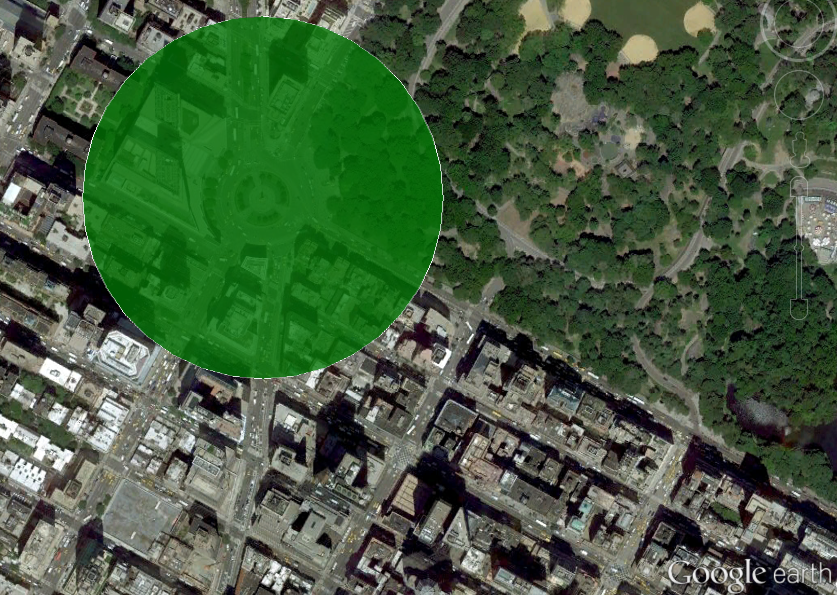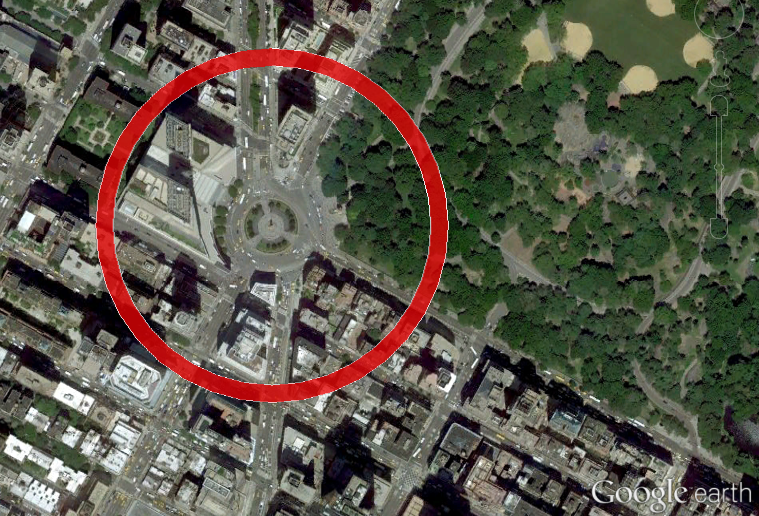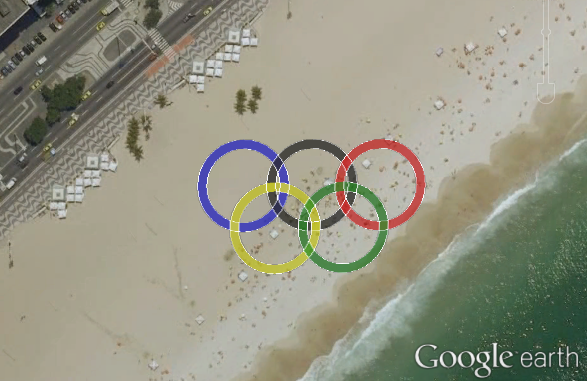# KML circles¶

## Basic KML circle¶

Generates a circle approximation readable by simpleKML.

```from polycircles import polycircles

polycircle = polycircles.Polycircle(latitude=40.768085,
longitude=-73.981885,
number_of_vertices=36)
kml = simplekml.Kml()
pol = kml.newpolygon(name="Columbus Circle, Manhattan",
outerboundaryis=polycircle.to_kml())
pol.style.polystyle.color = \
simplekml.Color.changealphaint(200, simplekml.Color.green)
kml.save("test_kml_polygon_3_manhattan.kml")
```

Note that a polygon with 36 vertices looks pretty much like a circle:A Polycircle above Columbus Square, Columbus Square, Manhattan, NYC, United States

## Donut! (Well, Torus)¶

Using the `innerboundaryis` of simpleKML Polygon object and two polycircles, a donut-shape can be easily created:

```outer_polycircle = polycircles.Polycircle(latitude=40.768085,
longitude=-73.981885,
number_of_vertices=36)
inner_polycircle = polycircles.Polycircle(latitude=40.768085,
longitude=-73.981885,
number_of_vertices=36)

kml = simplekml.Kml()
pol = kml.newpolygon(name="Torus around Columbus Circle, Manhattan",
outerboundaryis=outer_polycircle.to_kml(),
innerboundaryis=inner_polycircle.to_kml())
pol.style.polystyle.color = \
simplekml.Color.changealphaint(200, simplekml.Color.red)
kml.save("test_kml_polygon_2_torus_manhattan.kml")
```A Torus made from two Polycircles, Columbus Square, Manhattan, NYC, United States